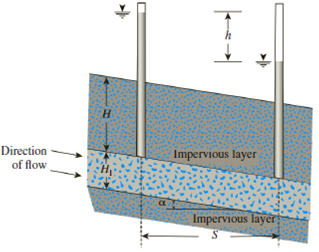Chapter 7, Problem 7.5PPrinciples of Geotechnical Enginee...

9th Edition
Braja M. Das + 1 other
ISBN: 9781305970939

Solutions

Chapter
SectionPrinciples of Geotechnical Enginee...

9th Edition
Braja M. Das + 1 other
ISBN: 9781305970939
Textbook Problem

A pervious soil layer is sandwiched between two impervious layers as shown in Figure 7.31. Find the rate of flow in m3/sec/m (at right angles to the cross section) through the pervious soil layer. Given: H = 3.5 m, H1 = 1.75 m, h = 2.5 m, S = 28 m, α = 12°, and k = 0.055 cm/sec.Figure 7.31

To determine

Find the rate of flow in m3/sec/m.

Explanation

Given information:

The height of the impervious layer H is 3.5 m.

The height of pervious soil layer H1 is 1.75 m.

The hydraulic conductivity of the permeable soil layer k is 0.055cm/sec.

The horizontal distance between the observation well S is 28 m.

The height difference in the water table h is 2.5 m.

The angle value α is 12°.

Calculation:

Determine the hydraulic gradient using the relation.

i=hScosα

Substitute 2.5 m for h, 28 m for S, and 12° for α.

i=2.528cos12°=0.0873

Determine the rate of flow using the relation.

q=kiA=ki(H1cosα×1)

Here, A is the area of the permeable layer

Still sussing out bartleby?

Check out a sample textbook solution.

See a sample solution

The Solution to Your Study Problems

Bartleby provides explanations to thousands of textbook problems written by our experts, many with advanced degrees!

Get Started

What is a milestone, and why is it significant to project planning?

Principles of Information Security (MindTap Course List)

What type of metals should not be ground on a pedestal grinder?

Precision Machining Technology (MindTap Course List)

How would you explode DFDs?

Systems Analysis and Design (Shelly Cashman Series) (MindTap Course List)

Describe residual risk.

Management Of Information Security

Describe the purpose of a pointing device. Give an example.

Enhanced Discovering Computers 2017 (Shelly Cashman Series) (MindTap Course List)